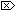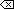The Open Group Base Specifications Issue 6
IEEE Std 1003.1-2001

NAME

fdim, fdimf, fdiml - compute positive difference between two floating-point numbers

SYNOPSIS

#include <math.h>

double fdim(double
x, double y);
float fdimf(float
x, float y);
long double fdiml(long double
x, long double y);

DESCRIPTION

[CX]The functionality described on this reference page is aligned with the ISO C standard. Any conflict between the requirements described here and the ISO C standard is unintentional. This volume of IEEE Std 1003.1-2001 defers to the ISO C standard.These functions shall determine the positive difference between their arguments. If x is greater than y, x- y is returned. If x is less than or equal to y, +0 is returned.

An application wishing to check for error situations should set errno to zero and call feclearexcept(FE_ALL_EXCEPT) before calling these functions. On return, if errno is non-zero or fetestexcept(FE_INVALID | FE_DIVBYZERO | FE_OVERFLOW | FE_UNDERFLOW) is non-zero, an error has occurred.

RETURN VALUE

Upon successful completion, these functions shall return the positive difference value.

If x- y is positive and overflows, a range error shall occur and fdim(), fdimf(), and fdiml() shall return the value of the macro HUGE_VAL, HUGE_VALF, and HUGE_VALL, respectively.

If x- y is positive and underflows, a range error may occur, and either ( x- y) (if representable), [XSI]or 0.0 (if supported),or an implementation-defined value shall be returned.

[MX]If x or y is NaN, a NaN shall be returned.ERRORS

The fdim() function shall fail if:

Range Error
The result overflows.

If the integer expression (math_errhandling & MATH_ERRNO) is non-zero, then errno shall be set to [ERANGE]. If the integer expression (math_errhandling & MATH_ERREXCEPT) is non-zero, then the overflow floating-point exception shall be raised.

The fdim() function may fail if:

Range Error
The result underflows.

If the integer expression (math_errhandling & MATH_ERRNO) is non-zero, then errno shall be set to [ERANGE]. If the integer expression (math_errhandling & MATH_ERREXCEPT) is non-zero, then the underflow floating-point exception shall be raised.

The following sections are informative.

None.

APPLICATION USAGE

On implementations supporting IEEE Std 754-1985, x- y cannot underflow, and hence the 0.0 return value is shaded as an extension for implementations supporting the XSI extension rather than an MX extension.

On error, the expressions (math_errhandling & MATH_ERRNO) and (math_errhandling & MATH_ERREXCEPT) are independent of each other, but at least one of them must be non-zero.

None.

None.